﻿ A Study on Anti Bipolar Q – Fuzzy Normal Semi GroupsPublications are Open
Access in this journal
Article Versions
Export Article
• Normal Style
• MLA Style
• APA Style
• Chicago Style
Research Article
Open Access Peer-reviewed

### A Study on Anti Bipolar Q – Fuzzy Normal Semi Groups

Journal of Mathematical Sciences and Applications. 2018, 6(1), 1-5. DOI: 10.12691/jmsa-6-1-1
Published online: January 09, 2018

### Abstract

In this manuscript, we present notions of anti bipolar Q - fuzzy normal semi groups and we present characterization of anti bipolar Q – fuzzy normal semi groups. Further, we have characterized a semi group that a semi lattice of groups in terms of anti bipolar Q – fuzzy ideals, generalized anti bipolar Q – fuzzy bi – ideals and anti bipolar Q – fuzzy quasi – ideals.

### 1. Introduction

The fundamental concept of bipolar valued fuzzy sets popularized by Lee 3. Bipolar valued fuzzy sets are an extension of fuzzy sets whose membership degree range is enlarged from the interval [0, 1] to [-1, 1]. In bipolar valued fuzzy set, the membership degree 0 means that elements are irrelevant to the corresponding property, the membership degree (0, 1] indicates that element some what satisfy the property and the membership degree [-1, 0) indicate that elements some what satisfy the implicit counter property, the study of bipolar fuzzy algebras has achieved great success, Many wonderful results have been obtained by some mathematical researchers, such as Yasodara and Sathappan 12, Shanmugapriya and Arjunan 8, 9, Uma Maheswari et al 11 and Massa'deh and Fora 5. On the other hand, in the pest several decades, studies on the subject of anti fuzzy ideals in semi groups introduced by Shabir and Nawaz 10. Hee et al 1 extended the concept of bipolar fuzzy set to semi groups theory, while Muthuraj et al 7, Khizar et al 2 Massa'deh 4 and Massa'deh and Al naser 6 extend the concept of bipolar fuzzy set to subring , hemi – ring and Γ- near rings. In this paper, we deal with anti bipolar Q – fuzzy sets to a semi groups and investigate related properties. Further, we discussed anti bipolar Q – fuzzy normal semi groups. We studied some characteristics of anti bipolar Q – fuzzy normal semi groups and discussed the some of its properties.

### 2. Preliminaries

Definition 2.1 5 Let X and Q be a non-empty arbitrary sets. A bipolar-valued Q- fuzzy set or bipolar fuzzy set μ in XQ is an object having the form μ = {(x, q), μ+ (x, q), μ(x, q) | x ∈ X q ∈Q}, where μ+ : XQ →[0, 1] and μ: XQ →[−1, 0] are mappings. The positive membership degree μ+ (x) denotes the satisfaction degree of an element x to the property corresponding to a bipolar-valued fuzzy set μ = {(x,q), μ+(x, q), μ(x, q) | x ∈ X q ∈Q}, and the negative membership degree μ(x, q) denotes the satisfaction degree of an element x to some implicit counter property corresponding to a bipolar-valued fuzzy set μ = {(x,q), μ+(x, q), μ(x, q) | x ∈ X q ∈Q }. If μ+ (x, q) ≠ (0, q) and μ(x, q) = (0, q), then it is the situation that x is regarded as having only positive satisfaction for μ = {(x,q), μ+(x, q), μ(x, q) | x ∈ X q ∈Q }. If μ+ (x, q) = (0, q) and μ(x, q) ≠ (0, q), then it is the situation that x does not satisfy the property of μ = {(x,q), μ+(x, q), μ(x, q) | x ∈ X q ∈Q }, but somewhat satisfies the counter property of μ = {(x,q), μ+(x, q), μ(x, q) | x ∈ X q ∈Q}. It is possible for an element x to be such that μ+ (x, q) ≠ (0, q) and μ(x, q) ≠ (0, q), when the membership function of property overlaps with its counter property over some portion of X. For the sake of simplicity, we shall use the symbol μ = (μ+, μ) for the bipolar-valued fuzzy set μ = {(x,q), μ+(x, q), μ(x, q) | x ∈ X q ∈Q}.

Definition 2.2 Let A be a subset of a semi group G and Q be an arbitrary set, then the complement characteristic function of A, that is χAc is defined byDefinition 2.3 A semi group G is called semi lattice of groups if it is the theoretical union of a family of mutually disjoint subgroups Gi ; i ∈ I such that for all i, j ∈ I the product Gi Gj are both contained in the same subgroup Gk (k ∈ I).

Definition 2.4 A semi group G is called Archimedean if, for all x, y ∈ G there exists a positive integer n such that xn ∈ GyG.

Definition 2.5 A semi group G is called weakly commutative if, for all x, y ∈ G there exists a positive integer n such that (xy) n ∈ yGy.

Definition 2.6 A bipolar Q – fuzzy subset μ of a semi group G is said to be anti bipolar Q- fuzzy sub – semi group of G if μ+(xy, q) ≤ max{μ+(x, q), μ+(y, q)} and μ-(xy, q) ≤ max{μ- (x, q), μ-(y, q)} for all x, y ∈ G and q∈ Q.

Definition 2.7 A bipolar Q – fuzzy subset μ of a semi group G is said to be anti bipolar Q- fuzzy left [right] ideal of G if μ+(xy, q) ≤ μ+(y, q) and μ-(xy, q) ≤ μ-(y, q) [μ+(xy, q) ≤ μ+(x, q) and μ-(xy, q) ≤ μ-(x, q)] for all x, y ∈ G and q∈ Q.

Definition 2.8 A bipolar Q – fuzzy subset μ of a semi group G is said to be anti bipolar Q- fuzzy ideal if it is both anti bipolar Q- fuzzy left ideal and anti bipolar Q- fuzzy right ideal of G.

Definition 2.9 A bipolar Q – fuzzy subset μ of a semi group G is said to be generalized anti bipolar Q- fuzzy bi - ideal of G if μ+(xyz, q) ≤ max{μ+(x, q), μ+(z, q)} and μ-(xyz, q) ≤ max{μ- (x, q), μ-(z, q)} for all x, y, z ∈ G and q∈ Q.

Definition 2.10 A bipolar Q – fuzzy sub- semi group μ of a semi group G is said to be anti bipolar Q- fuzzy bi - ideal of G if μ+(xyz, q) ≤ max{μ+(x, q), μ+(z, q)} and μ-(xyz, q) ≤ max{μ- (x, q), μ-(z, q)} for all x, y, z ∈ G and q∈ Q.

Definition 2.11 A bipolar Q – fuzzy subset μ of a semi group G is said to be anti bipolar Q- fuzzy quasi – ideal of G if μ ⊆ ( μ • λ) ∪ (λ • μ) , where λ is a bipolar Q – fuzzy subset that λ(x, q) = (0, q) for all x ∈ G and q∈ Q.

Proposition 2.12 For a semi group G, the following statements are equivalent

1. G is completely regular.

2. Every quasi ideal of G is semi prime.

3. Every bi – ideal of G is semi prime.

4. Every generalized bi – ideal of G is semi prime.

5. Every anti bipolar Q- fuzzy quasi – ideal is bipolar Q- fuzzy semi prime.

6. Every anti bipolar Q- fuzzy bi – ideal is bipolar Q- fuzzy semi prime.

7. Every anti bipolar Q- fuzzy generalized – ideal is bipolar Q- fuzzy semi prime.

8. For every anti bipolar Q – fuzzy quasi – ideal μ of G ∀ x ∈ G, q ∈ Q, μ(x, q) = μ(x2, q).

9. For every anti bipolar Q – fuzzy bi – ideal μ of G ∀ x ∈ G, q ∈ Q, μ(x, q) = μ(x2, q).

10. For every anti bipolar Q – fuzzy generalized bi – ideal μ of G ∀ x ∈ G, q ∈ Q, μ(x, q) = μ(x2, q).

Proof: Straight forward.

Lemma 2.13 For a semi group G, the following are equivalent

1. G is a semi lattice of groups.

2. Gis regular and xG= Gx for all x in G.

3. I1 ∩ I2 = I1. I2 for every left ideal I1 and every right ideal I2 of G.

4. G is regular and every one sided ideal G is a two sided of G.

Definition 2.14 A semi group G is said to be regular if for every element x ∈ G, there exists an element y ∈ G such that x = xyx or equivalently x ∈ xGx for every x ∈ G and H ⊆ HGH for every H ⊆ G.

Definition 2.15 A semi group G is said to be completely regular if xG= Gx for all x ∈ G.

Definition 2.16 A semi group G is said to be normal if x ∈ xGx and xG= Gx for every x ∈ G.

Proposition 2.17 Every weakly commutative semi group is a semi lattice of Archimedean semi groups.

### 3. Main Results

Definition 3.1 An anti bipolar Q – fuzzy quasi – ideal μ of a semi group G is called co – normal if μ(xy, q) = μ(yx, q) for all x, y ∈ G and q ∈ Q.

Definition 3.2 A semi group G is said to be anti bipolar Q – fuzzy anti co – normal if every anti bipolar Q – fuzzy quasi – ideal of G is co – normal.

Definition 3.3 An anti bipolar Q – fuzzy bi – ideal μ of a semi group G is called bi – normal if μ(xy, q) = μ(yx, q) for all x, y ∈ G and q ∈ Q.

Definition 3.4 A semi group G is said to be anti bipolar Q – fuzzy anti bi – normal if every anti bipolar Q – fuzzy bi – ideal of G is bi – normal.

Theorem 3.5 Let μ, δ be any anti bipolar Q- fuzzy bi – ideals of a semi group G, then their union is an anti bipolar Q – fuzzy bi – ideal of G.

Proof:

Sinceμ, δ be any anti bipolar Q- fuzzy bi – ideals of a semi group G and x, y ∈ G and q ∈ Q.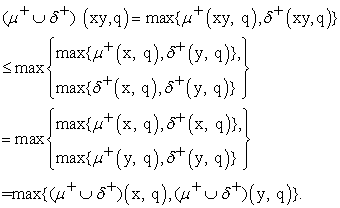Also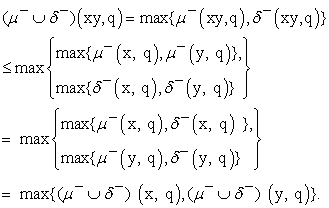Therefore μ ∪ δ is anti bipolar Q- fuzzy sub semi group of G.

On the other handAndWe get μ ∪ δ is anti bipolar Q- fuzzy bi - ideal of G.

Theorem 3.6 If G is a semi group that is a semi lattice of groups. Then every generalized anti bipolar Q – fuzzy bi – ideal of G is both anti bipolar Q – fuzzy ideal of G.

Proof:

Suppose that μ is a generalized anti bipolar Q – fuzzy bi – ideal of G and x, y ∈ G, then by Lemma 2.13 we have: xy ∈ (xGx)G = (xG)(Gx) = x(GG)x ⊆ xGx. Then there exist an element z ∈ G such that xy = xzx, thusμ +(xy, q) = μ +(xzx, q) ≤ max{μ +(x, q), μ +(x, q)}= μ +(x, q) and μ -(xy, q) = μ -(xzx, q) ≤ max{μ -(x, q), μ -(x, q)}= μ -(x, q), therefore μ is an anti bipolar Q – fuzzy right ideal of G. Similarly, we have μ is an anti bipolar Q – fuzzy left ideal of G and so μ is both anti bipolar Q – fuzzy ideal of G.

Theorem 3.7 Let G be a semi group, then G is semi lattice of groups iff every anti bipolar Q – fuzzy quasi – ideal μ of G; μ(x2, q) = μ(x, q) and μ(xy, q) = μ(yx, q) for all x, y ∈ G.

Proof:

(⇒) Since G is a semi lattice of a groups and μ is any generalized anti bipolar Q – fuzzy bi – ideal of G and x , y ∈ G. Then, by Lemma 2.13 G is regular, there exists z ∈ G such that x = xzx = (xzxzxzx) also by Lemma 2.13 xG = Gx, there exist u, v ∈ G such that zx = xu and xz = vx. Then we get x = xzx = x(zxzxzx) = x(zx)z(xz)x = x(xu)z(vx)x = x2(uzv)x2, on the other hand μ is generalized anti bipolar Q – fuzzy bi – ideal of G, we have:

μ +(x, q) =μ +( x2(uzv)x2, q) ≤ max{μ +(x2, q), μ +(x2, q)} = μ +(x2, q) = μ +( x(xzx), q) = μ +( x(xz)x, q) ≤ max{μ +(x, q), μ +(x, q)} = μ +(x, q) and so μ +(x, q) = μ +( x2, q) . Dually, we have that μ -(x, q) = μ -( x2, q) also by lemma 2.13, we have: (xy)4 ∈ (Gxy)G(yxG) = (yxG)G(Gyx) then there exist ∝ ∈ G such that (xy)4 = yx∝yx. Hence μ +(xy, q) = μ +((xy)2, q) = μ +((xy)4, q) = μ +((yx)∝(yx), q) ≤ max{μ +(yx, q), μ +(yx, q)}= μ +(yx, q). Thus μ +(xy, q) ≤ μ +(yx, q). Similarly, we have μ +(yx, q) ≤ μ +(xy, q) and so μ +(xy, q) = μ +(yx, q). By the same proof we get μ -(xy, q) = μ -(yx, q) and hence μ (xy, q) = μ (yx, q).

(⇐) Suppose that μ is an anti bipolar Q – fuzzy quasi – ideal of G such that μ(x2, q) = μ(x, q) and μ(xy, q) = μ(yx, q) for all x, y ∈ G and q ∈ Q. Then by Proposition 2.17that G is completely regular, if z ∈ G we need to show zG= Gz . Let zv ∈ zG ; v ∈ G then the complement characteristic function of A[vz] that is χA[vz]c is an anti bipolar Q – fuzzy quasi – ideal of G. Then by assumption, we have χA[vz]c(zv) = χA[vz]c(vz) = 0 and so zv ∈ A[zv] = {zv} ∪ (zvG ∩ Gzv). If zv = vz, then zv = vz ∈ Gz and so zG ⊆ Gz . If zv ∈ vzG, then zv ∈ vzG ∩ Gvz ⊆ Gvz ⊆ Gz and so zG ⊆ Gz therefore zG ⊆ Gz also Gz ⊆ zG. Then Gz = zG hence by Lemma 2.13 we get G is a semi lattice of groups.

Corollary 3.8 Let G be a semi group, then the following are equivalent

1. For every anti bipolar Q- fuzzy bi – ideal μ such that μ(x2, q) = μ(x, q) and μ(xy, q) = μ(yx, q) for all x, y ∈ G and q ∈ Q.

2. For every generalized anti bipolar Q- fuzzy bi – ideal μ such that μ(x2, q) = μ(x, q) and μ(xy, q) = μ(yx, q) for all x, y ∈ G and q ∈ Q.

Proof: Straightforward.

Proposition 3.9 If δ is anti bipolar Q-fuzzy anti co – normal semi group then its normal.

Proof:

Suppose that x ∈ δ and let xy ∈ xδ such that y ∈ δ. Then the complement characteristic function of A[yx], that is χA[yx]c is an anti bipolar Q – fuzzy quasi – ideal of δ, therefore it is anti bipolar Q – fuzzy normal. Since yx ∈ A[yx] we have χA[yx]c(xy) = χA[yx]c(yx) = 0 and so xy ∈ A[yx] = {yx} ∪ (yxδ ∩ δyx) ⊆ δx. Then xδ ⊆ δx, similarly we have δx ⊆ xδ therefore xδ = δx and we get δ is normal.

Theorem 3.10 Any anti bipolar Q – fuzzy anti bi – normal semi group is normal.

Proof:

Let δ be an anti Q – fuzzy anti bi – normal semi group and a ∈ δ such that xy ∈ xδ where y ∈ δ then the complement characteristic function of K[yx], that is χK[yx]c is an anti bipolar Q – fuzzy bi – ideal of δ. Therefore its anti bipolar Q – fuzzy bi – normal, since yx ∈ K[yx] and we have χK[yx]c(xy) = χK[yx]c(yx) = 0 and so xy ∈ K[yx] = {yx} ∪ {yxyx} ∪ (yxδ yx) ⊆ δx. Thus xδ ⊆ δx similarly δx ⊆ xδ and therefore xδ = δx, hence δ is normal.

Theorem 3.11 If δ is normal semi group of a group G, then δ is anti bipolar Q – fuzzy anti bi – normal semi group.

Proof:

Let μ be any anti bipolar Q – fuzzy bi – ideal of δ then δ is regular and normal, we have: xy ∈ (xδx)(yδy) = (xδ)(xy)(δy) ⊆ (xδ)(xyδxy)(δy) = (xδx)y(δx)(yδy) ⊆ (δy)(xδ)δ = δ (yx) δδ = (yx)δδδ ⊆ yxδ = (yxδyx)δ = (yxδ)(δyx) = yx(δδ)yx ⊆ yxδyx. Thus there exist z ∈ δ such that xy = yxzyx but μ is an anti bipolar Q – fuzzy bi – ideal of δ, we have :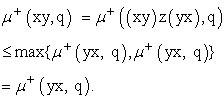Also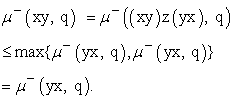We get μ (xy, q) ≤ μ (yx, q), similarly we get μ (xy, q) ≥ μ (yx, q) and therefore μ (xy, q) = μ (yx, q) so that δ is an anti bipolar Q – fuzzy anti bi – ideal.

Corollary 3.12 If δ is anti bipolar Q – fuzzy anti co – normal independent semi group of a group G, then δ is commutative.

Proof: Straight forward.

Theorem 3.13 If δ is an anti bipolar Q – fuzzy anti bi – normal semi group of a group G, then δ is a semi lattice of Archimedean semi groups.

Proof:

Let μ be any anti bipolar Q – fuzzy anti bi – normal semi group and let x, y ∈ δ, μ be any anti bipolar Q – fuzzy bi ideal of δ. Then the complement characteristic function of K[yx], that is χK[yx]c is an anti bipolar Q – fuzzy bi – ideal of δ. Therefore its anti bipolar Q – fuzzy bi – normal, yx ∈ K[yx] and we have χK[yx]c(xz) = χK[yx]c(zx) = 0 and so xy ∈ K[yx] = {yx} ∪ {yxyx} ∪ (yxδ yx) ⊆ δx. Thus xy ∈ yxδyx, then is weakly commutative and therefore by Proposition 2.17 that δ is semi lattice of Archimedean semi groups.

Theorem 3.14 If δ is an anti bipolar Q – fuzzy anti co – normal semi group of a group G, then it is a semi lattice of Archimedean semi groups.

Proof:

Let μ be any anti bipolar Q – fuzzy anti co – normal semi group and x, y ∈ δ, μ be an anti bipolar Q – fuzzy quasi ideal of δ. Then the complement characteristic function of L[yx], that is χL[yx]c is an anti bipolar Q – fuzzy quasi – ideal of δ. Thus its anti bipolar Q – fuzzy co – normal, since yx∈ L[yx] we have χL[yx]c(xz) = χL[yx]c(zx) = 0 and xy ∈ L[yx] = {yx} ∪ (yxδ ∩ δyx) ⊆ yxδ then by Proposition 3.9 we have: (xy)2 ∈ (yxδ)(yxδ) = (yxδ)(δyx) = (yx)δδ(yx) ⊆ yxδyx = y(xδy)x ⊆ yδx thus (xy)2 ∈ yδx and hence δ is weakly commutative, by Proposition 2.17 δ is a semi lattice of Archimedean semi groups.

Proposition 3.15 If δ is a completely regular semi group such that δ is anti bipolar Q – fuzzy anti Q – fuzzy anti bi – normal semi group, then δ is anti bipolar Q – fuzzy anti co – normal semi group.

Proof: straight for ward.

Corollary 3.16 Let δ be completely regular semi group such that δ be an anti bipolar Q – fuzzy anti co – normal, then for each x, y ∈ δ there exist n ∈ N such that (xy)n ∈ yxδyx.

Proof:

Since δ is an anti bipolar Q – fuzzy anti co – normal, then by Proposition 3.9 δ is normal. Let x, y ∈ δ then we have: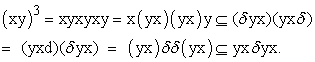Therefore (xy)3 ∈ yxδyx.

Corollary 3.17 Let δ be completely regular semi group such that for each x , y ∈ δ there exist n ∈ N such that (xy)n ∈ yxδyx.

Proof:

Let μ be any anti bipolar Q – fuzzy bi – ideal of δ and x, y ∈ δ, then there exist n ∈ N such that (xy)n = yxzyx but δ is completely regular then there exist w ∈ δ such that xy = (xy)n w(xy)n, μ is an anti bipolar Q – fuzzy bi – ideal of δ then we have:Similarly, we have μ + (xy, q) ≥ μ + (yx, q) and we get μ + (xy, q) = μ + (yx, q).

AndSimilarly, we have μ - (xy, q) ≥ μ - (yx, q) and we get μ - (xy, q) = μ - (yx, q). Therefore μ (xy, q) = μ (yx, q) and hence δ is anti bipolar Q – fuzzy anti bi – normal.

### 4. Conclusion

In this paper, we introduced the notion of anti bipolar Q – fuzzy ideal semi group that is a semi lattice of groups, generalized anti bipolar Q – fuzzy bi – ideals and anti bipolar Q – fuzzy quasi – ideals. Also, we introduce anti bipolar Q – fuzzy normal semi groups. In the next paper, we study properties of anti bipolar Q – fuzzy ideal, bi – ideal and normal homomorphism Acknowledgements

### Acknowledgements

Authors are highly thankful to the referees for has valuable suggestions.

### References

  Hee Young Ban., Myoung Jo Kim and Yoon Jungpark, Bipolar Fuzzy Ideals With Operators in Semi Groups. Annals of Fuzzy Mathematics and Informatics, Vol.4, No. 2(2012). pp. 253-265. In article View Article  Khizar Hayat, Tahir Mahmood and Bing – Yuan Cao, On Bipolar Anti Fuzzy h – Ideals in Hemi – Rings. Fuzzy Information and Engineering, Vol.9 (2017). pp. 1-19. In article View Article  Lee, K. M., Bipolar Fuzzy Sub Algebras and Their Operations. Proc. Int. Conf. On Intelligent Technologies, Bangkok, Thailand, pp. 307-312, (2000). In article PubMed  Massa'deh, M.O., On Bipolar Fuzzy Cosets, Bipolar Fuzzy Ideals and Homeomorphisms of Γ - Near Rings. Far East Journal of Mathematical Sciences (FJMS), Vol.102, No. 4(2017). pp. 731-747. In article  Massa'deh, M.O., and Fora, A.A., Bipolar – Valued Q – Fuzzy HX Subgroup on an HX Group. Journal of Applied Computer Science and Mathematics, Vol. 11, No. 23(2017). pp. 20-24. In article View Article  Massa'deh, M.O., and Al – Naser, A.M., Homomorphism in a Bipolar – Valued Q – Fuzzy Subring. Global Journal of Pure and Applied Mathematics, Vol.12, No.5(2016). pp. 4233-4241. In article  Muthuraj, R., Murhuraman, M.S and Rajinikannan, M., Bipolar Anti – Fuzzy Subrings. International Journal on Research Innovations in Engineering Science (IJRIEST), Vol.1, No.3 (2016). pp.16-21. In article View Article  Shanmugapriya, V., and Arjunan, K., Bipolar Valued Fuzzy Subsemiring of a Semiring Using Homomorphism and Anti – Homomorphism. International Journal of Mathematical Archive, Vol.7, No.12(2016). pp. 99-102. In article View Article  Shanmugapriya, V., and Arjunan, K., Some Translators in Bipolar Valued Fuzzy Subsemiring of a Semiring. Bulletin of Mathematics and Statistics Research, Vol.4, No.4(2016). pp. 118-123. In article  Shabir, M., and Nawaz, Y., Semi Groups Characterized By The Properties of Ideals. Journal of Advanced Research in Pure Mathematics, Vol. 3(2009). pp. 42-59. In article  Uma Maheswari, P., Arjunan, K., and Mangayarkarasi, R., Notes On Bipolar Valued Fuzzy Subrings of a Ring. International Journal of Applied Mathematical Sciences, Vol.9, No.1 (2016). pp. 89-97. In article View Article  Yasdara, B., and Sathappan, KE., Sub Semirings Bipolar – Valued Multi Fuzzy of a Semiring. International Journal of Mathematical Archive, Vol.6, No. 9(2015). pp. 75-80. In articleThis work is licensed under a Creative Commons Attribution 4.0 International License. To view a copy of this license, visit http://creativecommons.org/licenses/by/4.0/